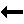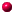What is this thing called Painlevé?contents ODEs and Singularities Consider the ordinary differential equation (ODE) $$y'=-y^2\qquad\qquad (0.1)$$ The solution is simple to find by separation of variables. Divide both sides by $$y^2$$ and integrate both sides. This leads to the solution $$y={1}/(x-a)$$ where $$a$$ is a free constant. This is a "general" solution (because it has one free constant, corresponding to the order of the equation). There is also a "special" solution: $$y=0$$. The solutions we have found are analytic except for the general solution at the point $$x=a$$. This point is movable! Its location changes with the initial condition that defines a particular solution. E.g., \begin{align} &y(0)=1 \Rightarrow a=-1\\ &y(0)=-2 \Rightarrow a=1/2. \end{align} Note that this movable singularity is a pole. Excercise: Solve the ODE$$y'=-y^3$$ and show that the general solution is multivalued around its movable singularities. Now, suppose we are given a different ODE $$y'=- y^2/x \qquad\qquad (0.2)$$ The general solution of Equation (0.2) is $$y=1/(\log(x)-a).$$ This solution has a logarithmic singularity at $$x=0$$ as well as a pole at $$x=\exp(a)$$. Notice that the first singularity, at $$x=0$$, does not change with initial conditions. It is fixed by the fact that $$x=0$$ occurs as a singularity in the equation itself. The property that all movable singularities of all solutions are poles is called the Painlevé property. Both ODEs (0.1) and (0.2) above have this property. Painlevé found the six equations now named after him by classifying second-order ODEs in a certain class which have this property.Back to ContentsNalini Joshi Last modified: 12 October 2011 by N.Joshi